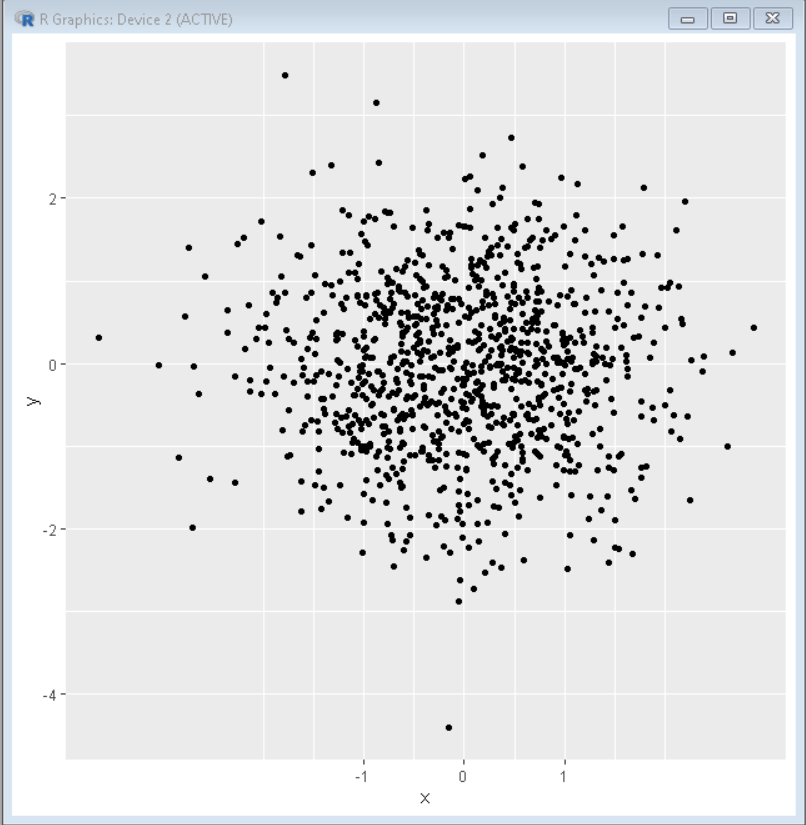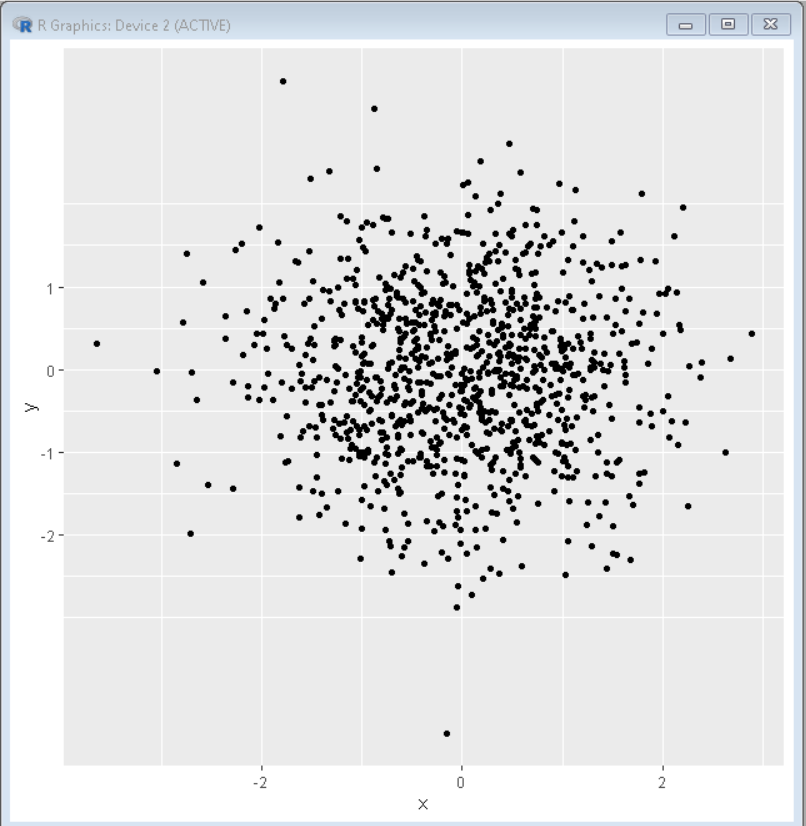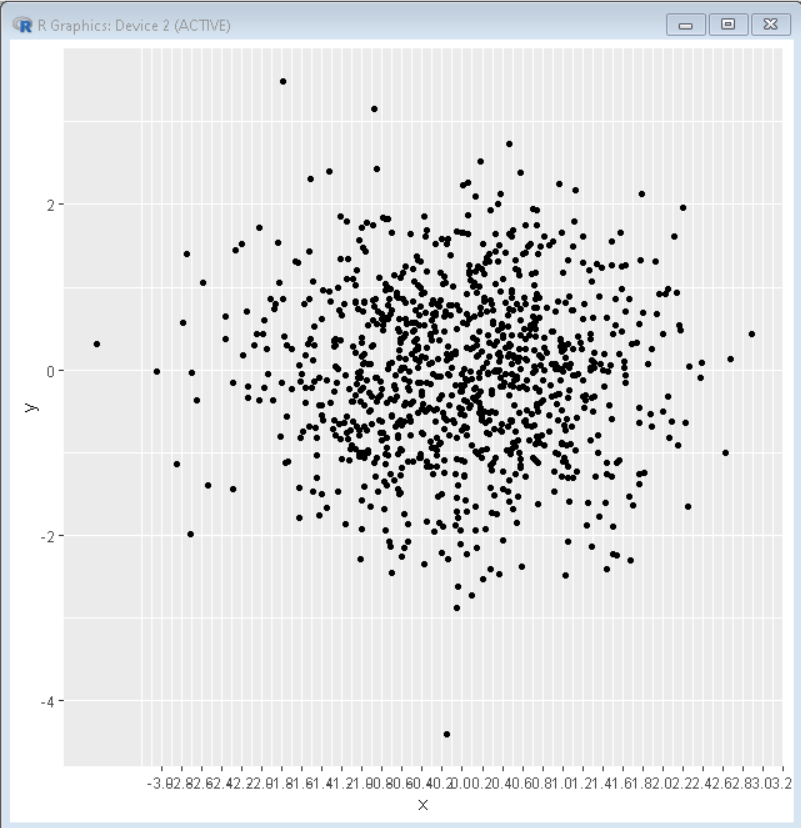Related Articles

# Set Axis Breaks of ggplot2 Plot in R

• Last Updated : 23 Aug, 2021

In this article, we are going to see how to set axis break of ggplot2 plot in R Programming Language.

To add axis breaks in ggplot2 plots in R, we use scale_x_break() and scale_y_break() functions. These functions take a vector as a parameter that has breakpoints.  If we need multiple breakpoints we can add those too.

Syntax:

scale_x_continuous(breaks = <break-vec>)

scale_y_continuous(breaks = <break-vec>)

### Example 1: Specify X-Axis Ticks in ggplot2 Plot

Here is a ggplot2 scatter plot with x-axis break using scale_x_continuous() function. This function has a breaks parameter that takes a vector as input which has all the points of axis break as vector points. So, here we can set the axis breaks point to a plot manually.

Code:

## R

 `# Create sample data``set.seed``(5642)   ``sample_data <- ``data.frame``(x = ``rnorm``(1000),        ``                    ``y = ``rnorm``(1000))``# Load ggplot2 and ggbreak``library``(``"ggplot2"``) ``library``(``"ggbreak"``) `` ` `# create plot with axis break``ggplot``(sample_data, ``aes``(x = x, y = y)) ``+ ``geom_point``() + ``scale_x_continuous``(breaks = ``c``(-1,0, 1))`

Output:### Example 2: Specify Y-Axis Ticks in ggplot2 Plot

Here is a ggplot2 scatter plot with y-axis break using the scale_y_continuous() function. This function has a breaks parameter that takes a vector as input which has all the points of y-axis break as vector points. So, here we can set the axis breaks point to a plot manually.

## R

 `# Create sample data``set.seed``(5642)   ``sample_data <- ``data.frame``(x = ``rnorm``(1000),        ``                    ``y = ``rnorm``(1000))``# Load ggplot2 and ggbreak``library``(``"ggplot2"``) ``library``(``"ggbreak"``) `` ` `# create plot with axis break``ggplot``(sample_data, ``aes``(x = x, y = y)) ``+ ``geom_point``() + ``scale_y_continuous``(breaks = ``c``(-2, -1, 0, 1))`

Output:### Example 3: Specifying Sequence of Axis Ticks in ggplot2 Plot

To specify the sequence of axis ticks we use the seq function as a parameter to breaks the property of scale_x_continuous / scale_y_continuous instead of vector. Here instead of giving input as a vector, we give input as a sequence that has three points, the first is starting break, the second is ending break and the third one is break period between starting and ending break.

Syntax: plot+ scale_x_continuous(breaks = <seq-vec>) / scale_y_continuous(breaks = <seq-vec>)

Code:

## R

 `# Create sample data``set.seed``(5642)   ``sample_data <- ``data.frame``(x = ``rnorm``(1000),        ``                    ``y = ``rnorm``(1000))`` ` `# Load ggplot2 and ggbreak``library``(``"ggplot2"``) ``library``(``"ggbreak"``) `` ` `# create plot with axis break``ggplot``(sample_data, ``aes``(x = x, y = y)) ``+ ``geom_point``() + ``scale_x_continuous``(breaks = ``seq``(-3, 4, 0.2))`

Output:My Personal Notes arrow_drop_up